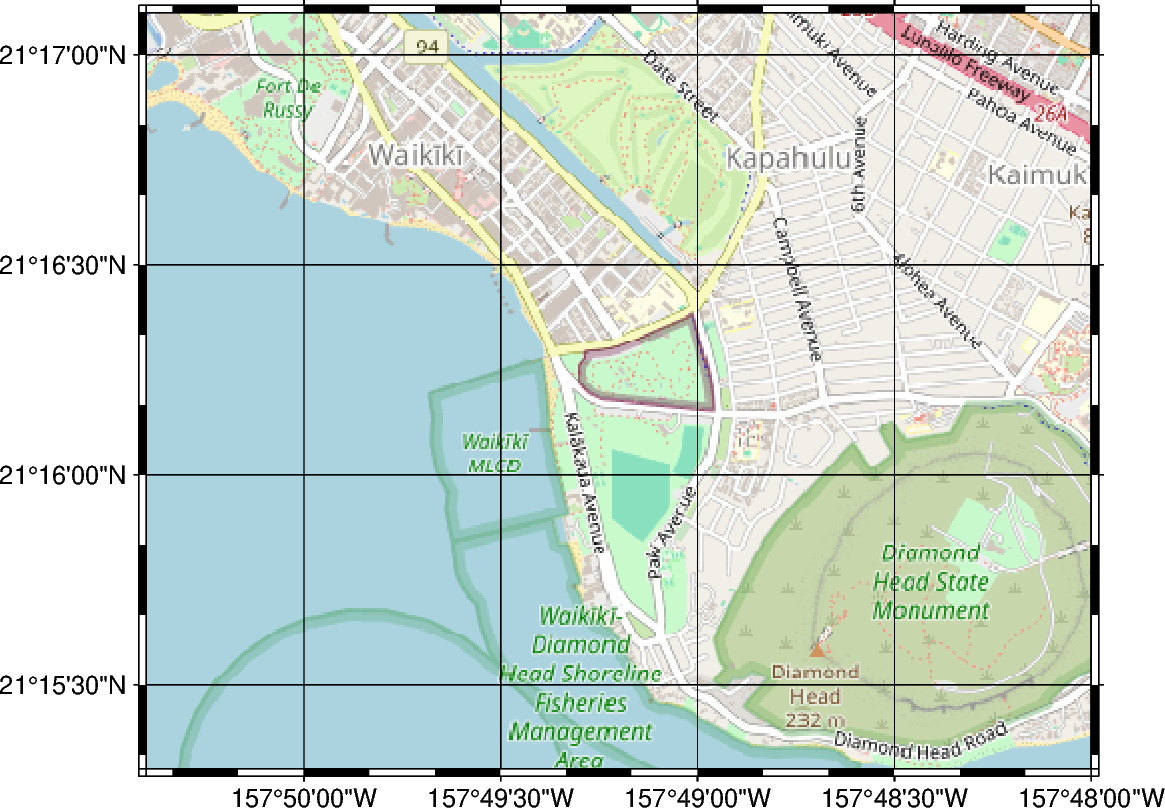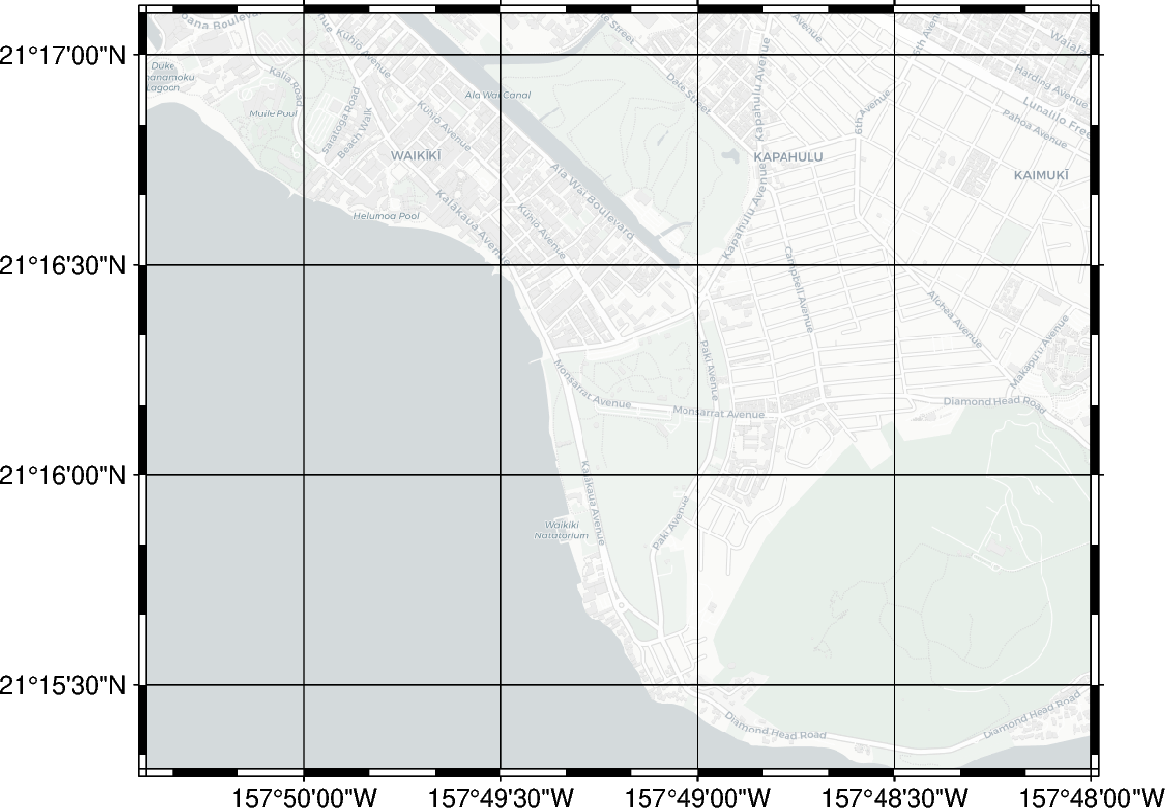# Tile maps

The `pygmt.Figure.tilemap` method allows to plot tiles from a tile server or local file as a basemap or overlay.

```import contextily
import pygmt

fig = pygmt.Figure()
fig.tilemap(
region=[-157.84, -157.8, 21.255, 21.285],
projection="M12c",
# Set level of details (0-22)
# Higher levels mean a zoom level closer to the Earth's
# surface with more tiles covering a smaller
# geographic area and thus more details and vice versa
# Please note, not all zoom levels are always available
zoom=14,
# Use tiles from OpenStreetMap tile server
source="https://{s}.tile.openstreetmap.org/{z}/{x}/{y}.png",
frame="afg",
)

fig.show()
``````grdimage [WARNING]: (w - x_min) must equal (NX + eps) * x_inc), where NX is an integer and |eps| <= 0.0001.
grdimage [WARNING]: w reset from -157.84 to -157.840070056
grdimage [WARNING]: (e - x_min) must equal (NX + eps) * x_inc), where NX is an integer and |eps| <= 0.0001.
grdimage [WARNING]: e reset from -157.8 to -157.799990801
grdimage [WARNING]: (s - y_min) must equal (NY + eps) * y_inc), where NY is an integer and |eps| <= 0.0001.
grdimage [WARNING]: s reset from 21.255 to 21.2549765742
grdimage [WARNING]: (n - y_min) must equal (NY + eps) * y_inc), where NY is an integer and |eps| <= 0.0001.
grdimage [WARNING]: n reset from 21.285 to 21.2850360149
```

It’s also possible to use tiles provided via the contextily library. See Contextily providers for a list of possible tilemap options.

```fig = pygmt.Figure()
fig.tilemap(
region=[-157.84, -157.8, 21.255, 21.285],
projection="M12c",
# Use the Stamen.Watercolor option from contextily
source=contextily.providers.Stamen.Watercolor,
frame="afg",
)

fig.show()
``````grdimage [WARNING]: (w - x_min) must equal (NX + eps) * x_inc), where NX is an integer and |eps| <= 0.0001.
grdimage [WARNING]: w reset from -157.84 to -157.840016369
grdimage [WARNING]: (e - x_min) must equal (NX + eps) * x_inc), where NX is an integer and |eps| <= 0.0001.
grdimage [WARNING]: e reset from -157.8 to -157.799990966
grdimage [WARNING]: (s - y_min) must equal (NY + eps) * y_inc), where NY is an integer and |eps| <= 0.0001.
grdimage [WARNING]: s reset from 21.255 to 21.2549741829
grdimage [WARNING]: (n - y_min) must equal (NY + eps) * y_inc), where NY is an integer and |eps| <= 0.0001.
grdimage [WARNING]: n reset from 21.285 to 21.2850347554
```

Total running time of the script: ( 0 minutes 4.232 seconds)

Gallery generated by Sphinx-Gallery